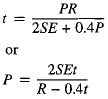Related Resources: pressure-vessel

### Thin Wall Pressure Vessels ASME Equation and Calculator

ASME Pressure Vessel Design and Engineering

ASME SECTION VIII - Thin Cylindrical Shells: Equations and Calculator:

The formulae in ASME Section VIII, Division 1, paragraph UG-27, used for calculating the wall thickness and design pressure of thin wall pressure vessels, are:

a) Circumferential Stress (longitudinal welds):
When, P < 0.385SE:Longitudinal Stress (circumferential welds):
When, P < 1.25SEWhere:
t = Minimum Design Wall Thickness (in);
P = Design Pressure (psi);
D = Tube Outside Diameter (in);
E = Welding Factor (1.0 for seamless pipe; 0.85 = for welded pipe);
C = Corrosion Allowance (0 for no corrosion; 0.0625 in. commonly used; 0.125 in. maximum);
S = Maximum Allowable Stress According to ASME Section II.